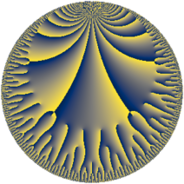# Properties

 Label 48.22.kLevel $48$ Weight $22$ Character orbit 48.k Rep. character $\chi_{48}(11,\cdot)$ Character field $\Q(\zeta_{4})$ Dimension $332$ Sturm bound $176$

# Related objects

## Defining parameters

 Level: $$N$$ $$=$$ $$48 = 2^{4} \cdot 3$$ Weight: $$k$$ $$=$$ $$22$$ Character orbit: $$[\chi]$$ $$=$$ 48.k (of order $$4$$ and degree $$2$$) Character conductor: $$\operatorname{cond}(\chi)$$ $$=$$ $$48$$ Character field: $$\Q(i)$$ Sturm bound: $$176$$

## Dimensions

The following table gives the dimensions of various subspaces of $$M_{22}(48, [\chi])$$.

Total New Old
Modular forms 340 340 0
Cusp forms 332 332 0
Eisenstein series 8 8 0

## Trace form

 $$332 q - 2 q^{3} - 4 q^{4} + 142760908 q^{6} - 8 q^{7} + O(q^{10})$$ $$332 q - 2 q^{3} - 4 q^{4} + 142760908 q^{6} - 8 q^{7} + 62460937496 q^{10} + 182904549904 q^{12} - 4 q^{13} - 23903738186800 q^{16} + 34734061105684 q^{18} + 46007763621428 q^{19} - 20920706408 q^{21} - 72242103936712 q^{22} - 1042071723098616 q^{24} - 1147739795850518 q^{27} - 2496568019593832 q^{28} - 8439084824218892 q^{30} - 4 q^{33} + 54581416306504072 q^{34} - 113121024435887644 q^{36} - 4 q^{37} - 113241280946212204 q^{39} - 175962409853129800 q^{40} - 123842746310914824 q^{42} + 353894423668227212 q^{43} - 953674316406252 q^{45} - 543256250318456744 q^{46} - 280111167033071568 q^{48} + 24576018019664496300 q^{49} + 2352404135975436504 q^{51} + 1106073080120792768 q^{52} + 2018503463813104040 q^{54} + 7927109680263600664 q^{55} + 3863696965439775416 q^{58} - 18295330336042015648 q^{60} - 10385362452353654068 q^{61} - 38933980587484296520 q^{64} + 123764601270055383268 q^{66} + 27307622196852988700 q^{67} + 20920706404 q^{69} - 29970527207468945480 q^{70} - 92584908768977075192 q^{72} - 29258275277343308674 q^{75} + 446519258070535329992 q^{76} + 55186286768870708700 q^{78} - 4 q^{81} + 174057355692587040960 q^{82} - 106130735006677337456 q^{84} + 323659102309414062496 q^{85} + 1529385670495185283140 q^{87} - 313703886119376940768 q^{88} + 1613345920711030853360 q^{90} + 258869294369761842568 q^{91} - 669717759523341595748 q^{93} - 1947195113041153735056 q^{94} - 3683062557381093425912 q^{96} - 8 q^{97} + 1098747022902677396108 q^{99} + O(q^{100})$$

## Decomposition of $$S_{22}^{\mathrm{new}}(48, [\chi])$$ into newform subspaces

The newforms in this space have not yet been added to the LMFDB.